From the images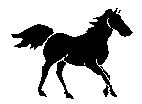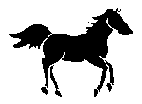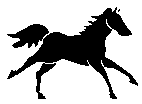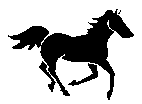generate these: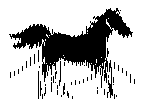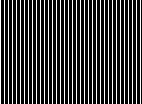Now moving the grid on the combined image will result in images like these: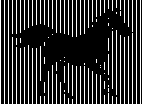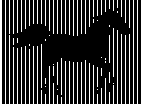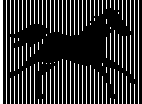```;; -*- mode: lisp; syntax: common-lisp -*-
;;
;; Peter Salvi, 2006.
;;
;; Animation Maker for 张济梅

;; File I/O

"Reads a decimal number from a stream that may have whitespace before it,
and _must_ have whitespace after it.
WARNING: This function also eats the first whitespace after the number."
(whitespace-list (mapcar #'char-code '(#\Space #\Tab #\Newline #\#)))
(result 0))
(loop while (member next whitespace-list) do
(when (= next (char-code #\#))
(loop until (= (read-byte stream) (char-code #\Newline))))
(loop until (member next whitespace-list) do
(setf result (+ (* result 10) (- next 48)))
result))

"Reads a PBM stream, and returns the contents as a two-dimensional array."
(unless (and (= (read-byte stream) (char-code #\P))
(error "Not a plain PBM stream"))
(result (make-array (list width height))))
(dotimes (j height)
(dotimes (i (ceiling width 8))
(dotimes (k 8)
(when (< (+ (* i 8) k) width)
(setf (aref result (+ (* i 8) k) j) (logbitp (- 7 k) c)))))))
result))

(defun write-raw-pbm (stream array)
"Writes the contents of ARRAY to a stream in raw (ASCII) PBM format."
(multiple-value-bind (sec min hrs day month year)
(get-decoded-time)
(format stream "P1~%~
# Generated by Animation Maker @ ~d-~d-~d ~d:~d:~d~%~
~d ~d~%"
year month day hrs min sec
(array-dimension array 0) (array-dimension array 1)))
(dotimes (j (array-dimension array 1))
(dotimes (i (array-dimension array 0))
(format stream "~:[0~;1~]" (aref array i j)))
(format stream "~%")))

(with-open-file (stream filename
:direction :input :element-type '(unsigned-byte 8))

;; Generating images

(defun generate-key (width height n)
"A pattern of vertical bars, with every nth being white."
(let ((result (make-array (list width height) :initial-element t)))
(dotimes (i (1+ (floor width n)))
(dotimes (j height)
(setf (aref result (* i n) j) nil)))
result))

(defun combine-pictures (lst)
"Combines N pictures by taking the Ith column from the (I mod N)th picture."
(let ((n (length lst))
(width (array-dimension (first lst) 0))
(height (array-dimension (first lst) 1))
(result (make-array (array-dimensions (first lst)))))
(dotimes (i width)
(dotimes (j height)
(setf (aref result i j) (aref (nth (mod i n) lst) i j))))
result))

(defun animate (output key &rest pictures)
"Convenience function for generating the `animated' and `key' image from
multiple original images."
(let ((n (length pictures))
(with-open-file (stream output :direction :output)
(write-raw-pbm stream combined))
(with-open-file (stream key :direction :output)
(write-raw-pbm stream (generate-key (array-dimension combined 0)
(array-dimension combined 1)
n)))))

;; Test